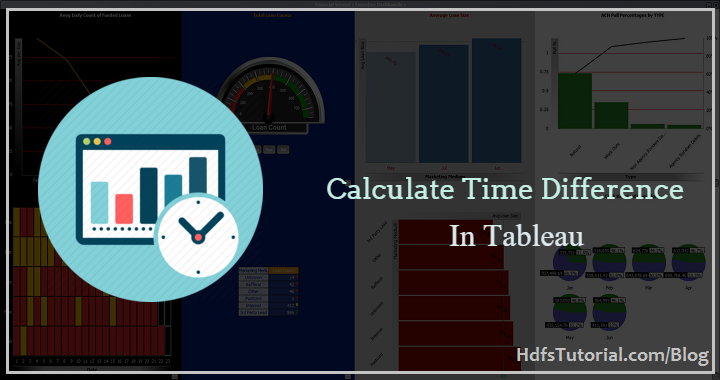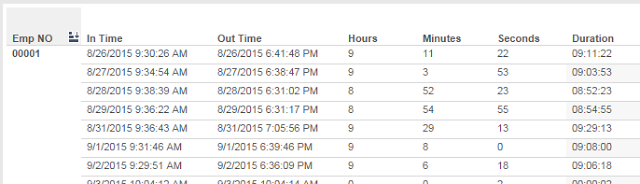Tableau

# How to Calculate The Time Difference In Tableau?

If you are working on Tableau production data, you must have come across the situation to calculate time difference in Tableau. Finding the date difference in the tableau is easy but the same is not with the time difference in Tableau.In this post, we will see how to calculate the time difference in Tableau using examples. We are writing this dedicated post to find time difference in Tableau because finding the difference between two time column in HH: MM: SS format needs some logical calculation.

To start with the methods to calculate the time differences in Tableau, let’s start with a simple example.

## Calculate Time Difference in Tableau

Here we will consider the following example-

Let’s say we have the biometric door data of certain employee working in an organization. Now we should find how much time the employee was at the work area.

The simple approach to do this is, check the in and out times of the door and find the difference of time.

The same we will be doing here. Let’s say below is the records for an employee-If you have to find only the difference in hours in Tableau, you could have easily done using the following formula-

DATEDIFF (‘hour’,[In Time],[Out Time])

Similarly, if you need to find the difference in minute, you can easily do using the following formula in tableau-

DATEDIFF(‘minute’,[In Time],[Out Time])

For the same data, if you need to calculate the difference in seconds then here is the formula-

DATEDIFF(‘second’,[In Time],[Out Time])

So, finding the difference for any one of the hour/minute/second is very easy but the same is not when you are finding time difference in tableau. Here you may have hours, minutes and seconds as well.

So, let’s see how you can calculate time difference in tableau-

Example: We have to find the difference between the following dates- 8/26/2015 9:30:26 Am and 8/26/2015 6:41:48 PM

### Step 1: Find difference in seconds

If you will find the difference in second using the following formula-

Difference in seconds:` `

`DATEDIFF('second',[In Time],[Out Time])`

It will result in 33,082 seconds.

### Step 2: Find the hour part from it

As 1 hour= 3600 seconds and so 33,082 seconds will have around 9.189 hours.

So, we will consider it to 9 hours and will take another remaining part in minute and seconds. But as it won’t be a manual stuff and so need to use the Tableau function.

For this, you can use Floor function of Tableau. Use the following formula to extract the hour from the second.

Floor([Duration in seconds]/3600)

This will result in you 9. But we need to get the result in HH format but here we have just a single digit. If you are thinking to append 0 using IF statement then it might hurt your performance.

Best way to do this is to use the STR function as shown below-

Right(STR(Floor([Duration in seconds]/3600)),2)

This will now give you 09 instead of 9.

### Step 3: Calculate the Minutes

As we had around 0.189 hours left and so this part must be converted into minutes and seconds.

To get remainder in tableau we have to use “Modulo” operator (%)

So the minute part can be found using-

`Right(STR(Floor(([Duration In Seconds]%3600)/60)),2)`

This will give you 11 which will be a minute part.

Now you have HH and MM part in place and you’re left with SS part. This can be found using the Modulo function.

### Step 4: Calculate the Second part

Using the following formula to find the second part-

`[Duration In Seconds]%60`

### Step 5: Make it to HH:MM:SS format

Now all you have to do is, just concatenate the results you have found in above steps as below.

[Hours] +’:’+[Minutes]+’:'[Seconds]

And you are done!

#### Conclusion

This was the easiest method to calculate time difference in Tableau. By this method you can easily find the time difference in HH:MM:SS format and use it further. Also, this is one of the top Tableau Interview Questions and so you should prepare for it.

Do try this and let us know if you will find any issue with it. If you liked this – How to calculate time difference in tableau, please share it with others. Also, please sign up for the freebies.

Note: If you’re new to Tableau and looking for Tableau training, you should browse to Tableau Online Training

### 1 Comment

•Imran Idris Shaikh says:

hours & Munites CF are becoming String Fields and seconds int filed and while combining them together getting error str & int values can’t be added